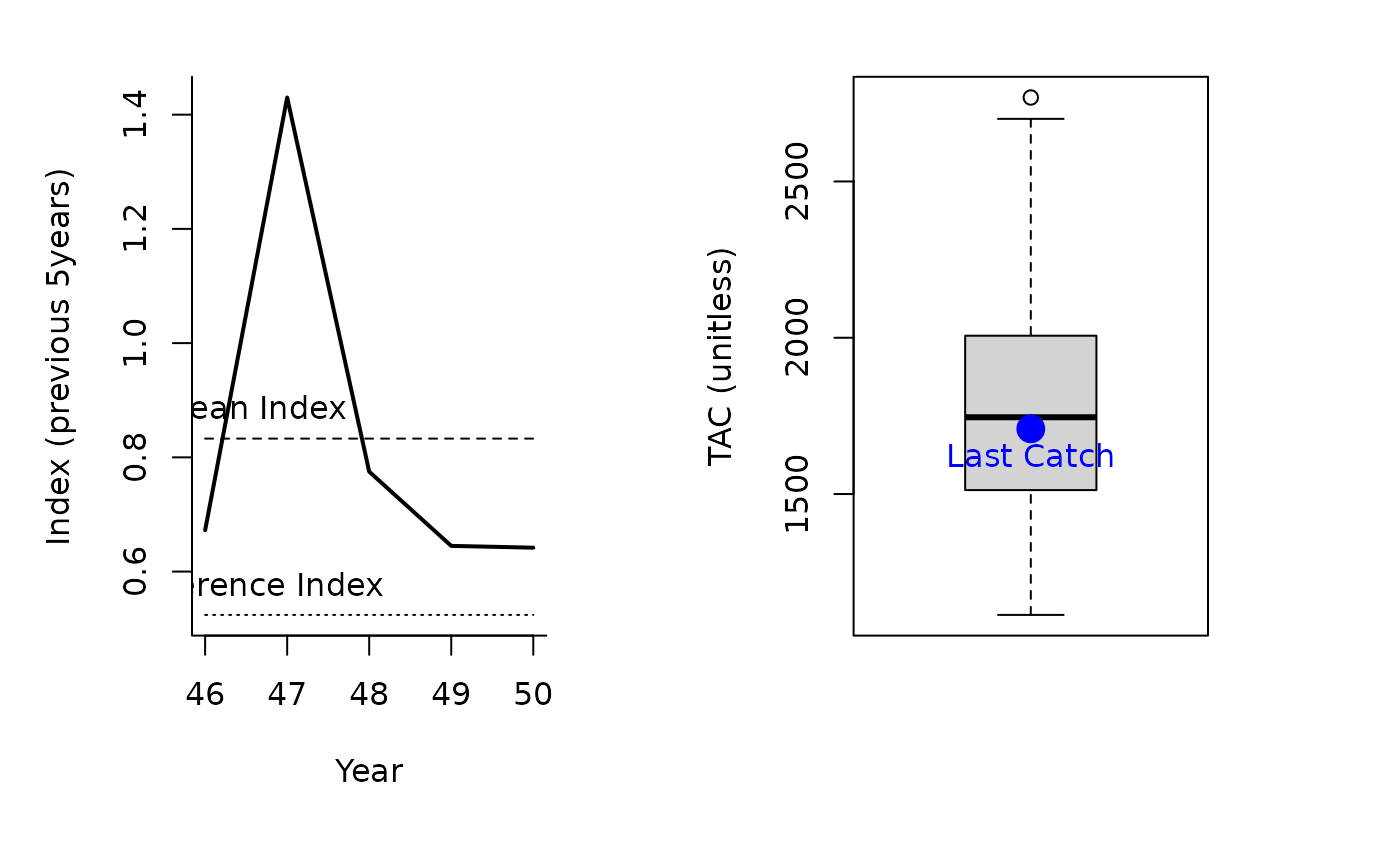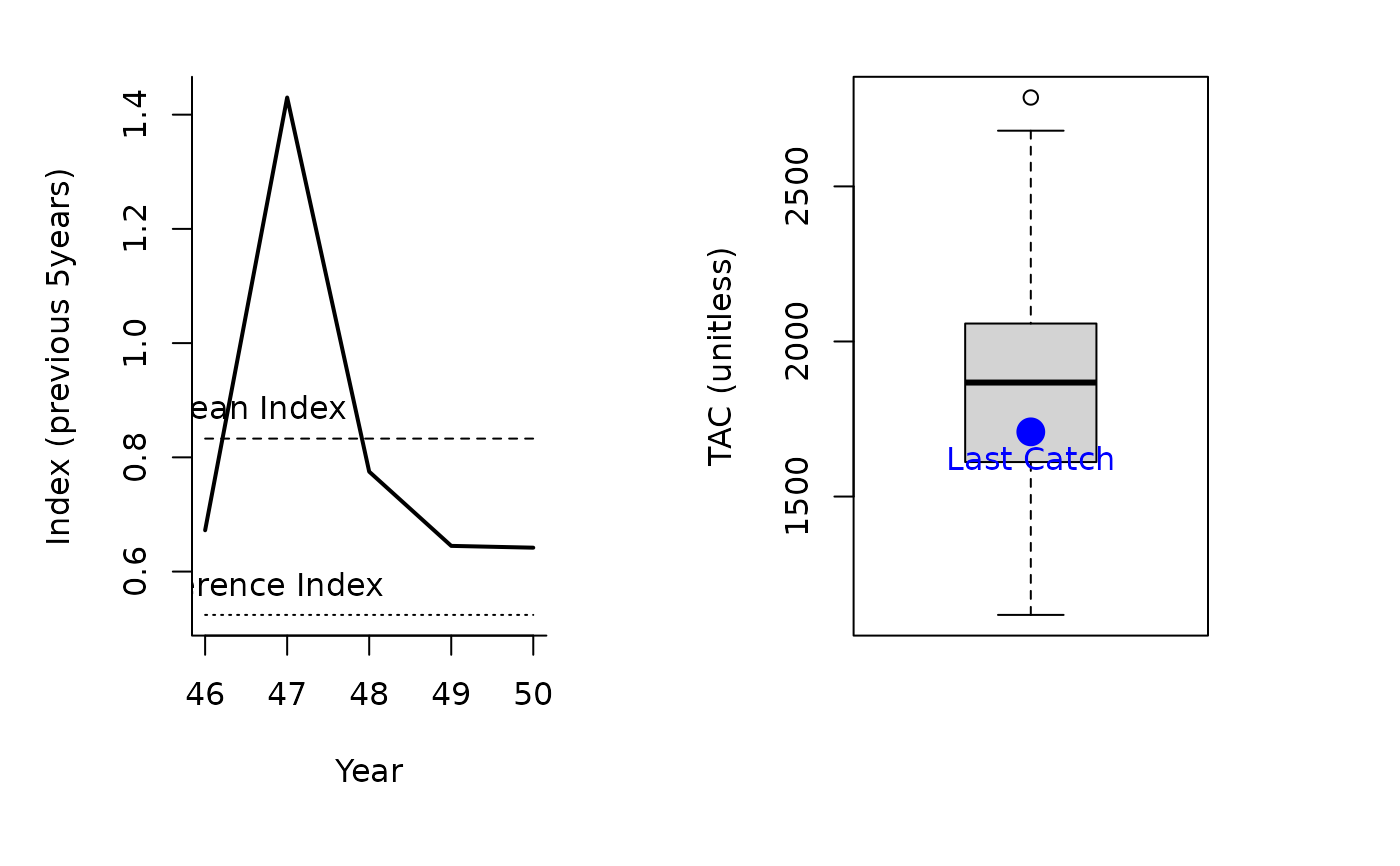An index target MP where the TAC is modified according to current index levels (mean index over last 5 years) relative to a target level.

IT5(x, Data, reps = 100, plot = FALSE, yrsmth = 5, mc = 0.05)

IT10(x, Data, reps = 100, plot = FALSE, yrsmth = 5, mc = 0.1)

## Arguments

x

A position in the data object

Data

A data object

reps

The number of stochastic samples of the MP recommendation(s)

plot

Logical. Show the plot?

yrsmth

The number of historical years over which to average the index

mc

The maximum fractional change in the TAC among years.

## Value

An object of class Rec-class with the TAC slot populated with a numeric vector of length reps

## Details

The TAC is calculated as: $$\textrm{TAC}_y = C_{y-1} I_\delta$$ where $$C_{y-1}$$ is the catch from the previous year and $$I_\delta$$ is the ratio of the mean index over the past yrsmth years to a reference index level. The maximum allowable change in TAC is determined by mc: e.g mc=0.05 means that the maximum change in TAC from the previous catch is 5%.

The reference index level (Data@Iref) is assumed to be a proxy for MSY. In the MSE Iref is the index at MSY subject to observation error (Obs@Irefbiascv). Consequently the performance of these methods in MSE is strongly determined by the uncertainty the in reference index.

## Functions

• IT5: Maximum annual changes in TAC are 5 per cent.

• IT10: Maximum annual changes are 10 per cent.

## Required Data

See Data-class for information on the Data object

IT5: Ind, Iref, MPrec

IT10: Ind, Iref, MPrec

## Rendered Equations

See Online Documentation for correctly rendered equations

T. Carruthers

## Examples

IT5(1, MSEtool::SimulatedData, plot=TRUE)#> TAC (median)
#>       1783.1
IT10(1, MSEtool::SimulatedData, plot=TRUE)#> TAC (median)
#>      1824.35